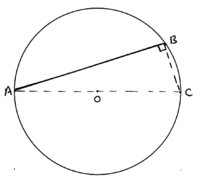# diameter and area of a circle

#### X049

##### New member
so i know that the diameter can divide a circle in two equal pieces (they have the same area) but what is the the mathematical proof to that?

#### topsquark

##### Senior Member
so i know that the diameter can divide a circle in two equal pieces (they have the same area) but what is the the mathematical proof to that?
It's one definition of the diameter. You know it's not a diameter if the area of the two parts aren't equal.

-Dan

#### lev888

##### Senior Member
so i know that the diameter can divide a circle in two equal pieces (they have the same area) but what is the the mathematical proof to that?
What's the formula for the area in question? It should point you to a proof.

#### pka

##### Elite Member
so i know that the diameter can divide a circle in two equal pieces (they have the same area) but what is the the mathematical proof to that?
The diameter of a circle is a line segment having its endpoints on the circle and which contains the center of the circle. A diameter divides the circle into two arcs of equal length. Because the center divides a diameter is two line segments of equal length, that being the radius, $$r$$ of the circle. It is well known that the area of the circle is $$\pi r^2$$ square units. Also well is the the length of the circumference of the circle is $$\pi D=2\pi r$$.
So the fact is that a diameter divides a circle into two areas of equal measures results from a diameter passing through the center.

•topsquark

#### Dr.Peterson

##### Elite Member
so i know that the diameter can divide a circle in two equal pieces (they have the same area) but what is the the mathematical proof to that?
There are various ways you might prove this formally, depending on what theorems you have about congruent sectors, or something like that.

But it's quite mathematical just to observe the symmetry of the figure. If you rotate it 180 degrees about the center, the resulting figure is identical; so you can conclude that the two "halves", each of which coincide with the other after the rotation, are congruent, and therefore have the same area.

•topsquark

#### lex

##### Full Member
What you have to do, depends on the definition you use.
The diameter (length) of a closed bounded shape like a circle, is the maximum of the distances between any two points on the shape.
If we take that as the definition of the diameter of a circle, then we can try to prove that the area is bisected by a diameter line.
Consider a diameter line AB, to be a line between 2 points A, B on the circle, which has that maximum, diameter length, d. We might prove that AB goes through the centre of the circle.
Suppose it does not. Then we can draw a triangle ABC:with BC≠0.
It is easily proved that the angle ABC is 90º
Now [MATH]AC^2=AB^2+BC^2[/MATH][MATH]\implies[/MATH] [MATH]AC>AB[/MATH] (since[MATH] BC≠0[/MATH])
Since A, C are points on the circle and AB is a diameter line of length d, this contradicts the fact that d is the maximum distance between 2 points on the circle.
Therefore, any diameter line goes through the centre.
Now you can use e.g. Dr.Peterson's symmetry argument, to show that the area of the circle is bisected by the diameter line.